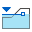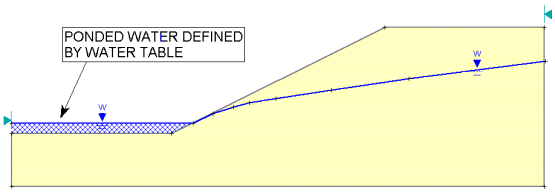The Rocscience International Conference 2021 Proceedings are now available. Read Now

## Search Results

A Water Table (phreatic surface) can be used for calculating pore pressure when the Groundwater Method in Project Settings is set to Water Surfaces. The Water Table can also be used to define ponded water above the slope surface.

1. Select Add Water Tablefrom the toolbar or the Boundaries menu.
2. Enter the vertices of the Water Table as described in Entering Coordinates.
3. IMPORTANT NOTE: the Water Table MUST BE DEFINED ACROSS ALL MATERIALS for which pore pressure is to be calculated using the Water Table. If it is not, then the analysis will not be able to calculate the pore pressure for slip surfaces where the Water Table is not defined, and a safety factor will NOT BE CALCULATED. Therefore, always make sure that the Water Table spans all relevant material zones of the model, or the slope will not be analyzed where the Water Table is undefined

4. When all vertices are entered, right-click and select Done, or press Enter with nothing in the prompt line.
5. You will now see the Assign Water Table dialog. Select the checkbox for each material for which pore pressure is to be calculated using the Water Table (by default all checkboxes are automatically selected.) NOTE:
• The Water Table can also be assigned to materials using the Define Material Properties dialog. The Assign Water Table dialog is simply a shortcut, which allows you to quickly assign the Water Table to all desired materials at once.
6. When you are finished with the Assign Water Table dialog, select OK.
7. The Water Table will appear on the model as a blue line. The first and last segments of the Water Table are marked by a triangular water level symbol, and a letter W. (The letter W is to distinguish the Water Table from Piezometric Lines, if they are also being used. Piezometric Lines are automatically numbered, starting at 1).
8. NOTE: only ONE Water Table can be defined for a model. After a Water Table is added, the Add Water Table option is disabled. If you want to add a completely new Water Table, then you must first delete the existing Water Table with the Delete Boundary option, and then add a new Water Table.

## Pore Pressure Calculation

For details about how pore pressure is calculated in Slide2 using a Water Table (or a Piezometric Line), see the Water Parameters topic in the Properties section of this Help system.

Ponded Water Defined By A Water Table

An important feature of the Water Table in Slide2, is the automatic creation of "ponded water", if the Water Table is drawn above the slope (External Boundary).

If a Water Table is drawn above the External Boundary, Slide2 will automatically create a region of ponded water below the Water Table and above the External Boundary. In general, this is the simplest and recommended way to model ponded water. The region of ponded water is automatically filled with a blue hatch pattern, as illustrated below.

Ponded water automatically created by Water TablePonded water affects the slope stability calculations, by the weight of the water on the slope (vertical force), and also by the horizontal hydrostatic force exerted on the slope. Also note:

9. If the setting of the Groundwater Method in Project Settings is:
• Ru Coefficients, or
10. then Ponded Water can still be defined by a Water Table, but the Water Table will NOT be used to calculate the pore pressure.

11. If the setting of the Groundwater Method in Project Settings is:
• Steady State FEA (finite element analysis)
12. then Ponded Water CANNOT be defined by a Water Table (the Add Water Table option will be disabled). When you are performing a finite element groundwater analysis, Ponded Water is automatically defined by the Total Head boundary conditions.

13. Ponded water can also be modeled as a "No Strength" material. In this case, the region of ponded water is included WITHIN the External Boundary, in the same manner as a soil region. A "No Strength" material must then be defined in the Define Materials dialog, and assigned to the region of ponded water. This is illustrated in the Strength Type topic. In most cases this is NOT recommended because it is much easier to model Ponded Water using a Water Table, than as a No Strength material.

## Saturated Unit Weight and the Water Table

If you wish to define different unit weights for your materials above and below a Water Table, then the Water Table must exist for this purpose.

• If a Water Table exists, then you may define both Saturated and Unsaturated Unit Weight of materials, in the Define Material Properties dialog.
• If a Water Table does not exist, then only a single unit weight can be defined for a material.

## Piezometric Lines vs. the Water Table

The method of pore pressure calculation is exactly the same for a Water Table or a Piezometric Line. However, Piezometric Lines are differentiated from the Water Table in Slide2 for the following reasons:

• The Water Table can be used to define ponded water, by simply drawing the Water Table above the External Boundary. This is illustrated above. A Piezometric Line drawn above the External Boundary, will NOT define ponded water.
• A Water Table allows you to define different Unit Weights for a material above and below the Water Table. This cannot be done with a Piezometric Line.
• A Water Table can be used in conjunction with a Pore Pressure grid, to ensure that pore pressures above the Water Table are always zero. (See the Water Pressure Grid topic for details.) Piezometric Lines cannot be defined in conjunction with Water Pressure Grids in Slide2.

## Rapid Drawdown Analysis

If you are performing a rapid drawdown analysis:

• The Add Water Table option is used to define the initial water level. In this case the water table is labeled W (Initial).
• You can define a second water table which defines the drawdown water level using the Add Drawdown Line option. A drawdown line is labeled W (Final).